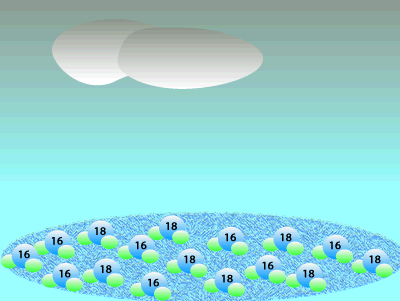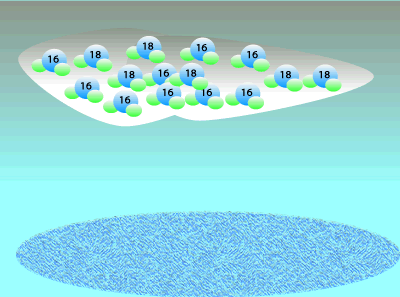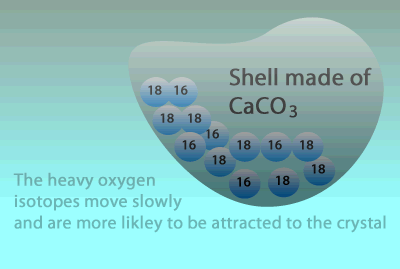Oxygen 16O and paleoclimatology How do we know what the Earth's temperature was millions of year ago? The isotopic composition of minerals formed in the past and of water trapped in glaciers gives us an indication of the average temperature during which the ice was deposited or minerals were formed. In fact, it is the oxygen isotope composition of ice and mineral deposit that is of immense interest. Oxygen consists of three isotopes: 16O, 17O, and 16O. A large percentage ((99.762%) of the Earth's oxygen is 16O and the rest we can say is 18O. A tiny, negligible, amount is 17O but we will ignore this for simplicity. Although the percentage of 18O is small we can still measure the ratio of 18O to 16O. This means that any mineral containing oxygen, or ice, will have a measurable ratio of 18O to 16O atoms. This ratio of the 18O to 16O is temperature dependent so we can determine the temperature at the time by simply measuring the ratio of these two isotopes. The 16O isotope is lighter than the 18O and so water molecules with the 16O isotope travel slightly faster at the same temperature than the water molecules with the heavier isotope. Because of its slightly greater speed it is easier to evaporate the lighter water molecule and so we find that a greater proportion of the lighter molecules will vaporise compared to the heavier molecules.When water vapour condenses to form rain it is the heavier, less mobile water molecules that form rain. This leaves a greater percentage of the lighter water molecule in the air as water vapour.The animation below shows how glacial ice forms with a relatively higher percentage of 16O than 18O. As water evaporates from the oceans 16O is concentrated in the atmosphere. As water vapour condensed to form rain the heavier isotope is removed from the atmosphere as rain leaving an even greater percentage of 16O in the air. As the atmospheric water vapour remaining travels over the poles it freezes to form glaciers.So, as water vapour travels toward the poles, it is increasingly enriched in 16O and depleted of 18O, the extent to which this happens depends on the temperature over the poles. In this case, colder temperatures lead to a greater 18O depletion. 1) What is an isotope? 2) What do isotopes have in common? 3) Two water molecules are at the same temperature, they have the same average kinetic energy. One has an 16O isotope while the other has an 18O isotope. Which one travels with greater speed? Explain. 4) In order to evaporate water molecules must break forces of attraction that act between water molecules in the liquid state. Fast moving molecules break their bonds easier than slow moving molecules. Explain why clouds are enriched with 16O isotope. 5) Condensation occurs when water molecules slow down so that forces of attraction take hold and bind water molecules together in the liquid state. Explain why rain is enriched with 18O isotope. 6) Explain how glaciers have a greater percentage of 16O than sea water found in the equator. 7) How can the temperature of a region that prevailed millions of years ago be estimated using oxygen isotopes? Solutions When forming minerals, soluble salts attract each other and precipitate out of solution thus forming solid crystals. Calcium carbonate is the main building material for marine animals' shells. A soluble mineral containing the heavier 18O isotope moves slower than a mineral containing the 16O isotope. Slower movement enables the heavier mineral to be trapped in the crystal structure where it is embedded in the shell of marine organisms.We can therefore use oxygen isotope ratio from fossils as well as from glacial ice to work out how global climate has changed over millions of years. We can thus make a few generalisations when looking at the information aobve. 1. Greater percentage of 18O isotopes are present in a mineral as the temperature in the area the mineral is formed gets lower. 2. The more glaciers that are formed the greater the percentage of 18O present in the oceans and so the greater the percentage of 18O in marine minerals. 3. The percentage of 18O decreases in glacial ice as the temperature also decreases. What impact would a colder world temperature have on the percentage of 18O in fossil deposits? Explain why glaciers formed from water vapour in the polar regions become 18O depleted as the climate cools while marine fossils become 18O enriched.